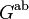# Perfect implies natural mapping from tensor square to exterior square is isomorphism

## Statement

For any group$G$, there is a natural surjective homomorphism from its tensor square to its exterior square:$G \otimes G \to G \wedge G$

defined as follows: it sends a generator of the form$g \otimes h$ to the corresponding generator$g \wedge h$.

The claim is that if$G$ is a perfect group, then this surjective homomorphism is also injective, i.e., its kernel is trivial, and hence, it is an isomorphism.

## Facts used

1. Exact sequence giving kernel of mapping from tensor square to exterior square: The exact sequence is:$H_3(G;\mathbb{Z}) \to \Gamma(G^{\operatorname{ab}}) \to G \otimes G \to G \wedge G \to 0$

## Proof

The proof follows from Fact (1), and the observation that if$G$ is perfect, then the abelianization$G^{\operatorname{ab}}$ is the trivial group, hence the universal quadratic functor applied to that also gives the trivial group. Thus, the exact sequence becomes:$H_3(G;\mathbb{Z}) \to 0 \to G \otimes G \to G \wedge G \to 0$

Because the mapping$G \otimes G \to G \wedge G$ is sandwiched by zero groups on both sides, it must be an isomorphism.